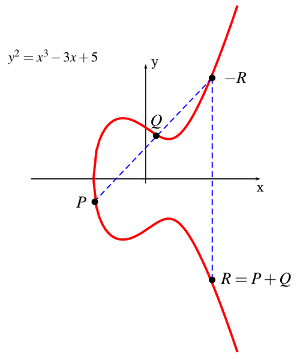### Elliptic Curve Cryptography (ECC)

In general, public-key cryptography systems use hard-to-solve problems as the basis of the algorithm. The most predominant algorithm today for public-key cryptography is RSA, based on the prime factors of very large integers. While RSA can be successfully attacked, the mathematics of the algorithm have not been comprised, per se; instead, computational brute-force has broken the keys. The defense is “simple” — keep the size of the integer to be factored ahead of the computational curve!

In 1985, Elliptic Curve Cryptography (ECC) was proposed independently by cryptographers Victor Miller (IBM) and Neal Koblitz (University of Washington). ECC is based on the difficulty of solving the Elliptic Curve Discrete Logarithm Problem (ECDLP). Like the prime factorization problem, ECDLP is another “hard” problem that is deceptively simple to state: Given two points, P and Q, on an elliptic curve, find the integer n, if it exists, such that P = nQ.

Elliptic curves combine number theory and algebraic geometry. These curves can be defined over any field of numbers (i.e., real, integer, complex) although we generally see them used over finite fields for applications in cryptography. An elliptic curve consists of the set of real numbers (x,y) that satisfies the equation:

y2 = x3 + ax + b

The set of all of the solutions to the equation forms the elliptic curve. Changing a and b changes the shape of the curve, and small changes in these parameters can result in major changes in the set of (x,y) solutions.#### FIGURE 19: Elliptic curve addition.

Figure 19 shows the addition of two points on an elliptic curve. Elliptic curves have the interesting property that adding two points on the elliptic curve yields a third point on the curve. Therefore, adding two points, P and Q, gets us to point R, also on the curve. Small changes in P or Q can cause a large change in the position of R.

So let’s go back to the original problem statement from above. The point Q is calculated as a multiple of the starting point, P, or, Q = nP. An attacker might know P and Q but finding the integer, n, is a difficult problem to solve. Q (i.e., nP) is the public key and n is the private key.

ECC may be employed with many Internet standards, including CCITT X.509 certificates and certificate revocation lists (CRLs), Internet Key Exchange (IKE), Transport Layer Security (TLS), XML signatures, and applications or protocols based on the cryptographic message syntax (CMS). RFC 5639 proposes a set of elliptic curve domain parameters over finite prime fields for use in these cryptographic applications and RFC 6637 proposes additional elliptic curves for use with OpenPGP.

RSA had been the mainstay of PKC for over a quarter-century. ECC, however, is emerging as a replacement in some environments because it provides similar levels of security compared to RSA but with significantly reduced key sizes. NIST use the following table to demonstrate the key size relationship between ECC and RSA, and the appropriate choice of AES key size:

TABLE 4. ECC and RSA Key Comparison.
(Source: Certicom, NIST)
ECC Key Size RSA Key Size Key-Size
Ratio
AES Key Size
163 1,024 1:6 n/a
256 3,072 1:12 128
384 7,680 1:20 192
512 15,360 1:30 256
Key sizes in bits.

Since the ECC key sizes are so much shorter than comparable RSA keys, the length of the public key and private key is much shorter in elliptic curve cryptosystems. This results into faster processing times, and lower demands on memory and bandwidth; some studies have found that ECC is faster than RSA for signing and decryption, but slower for signature verification and encryption.

ECC is particularly useful in applications where memory, bandwidth, and/or computational power is limited (e.g., a smartcard) and it is in this area that ECC use is expected to grow. A major champion of ECC today is Certicom; readers are urged to see their ECC tutorial.

Source: http://www.garykessler.net/library/crypto.html#ecc

SHARE